Next: The Adiabatic Approximation Up: Initial Conditions on the Previous: Initial Conditions in One

## The Initial value of the Hubble Constant

The initial value of the Hubble constant is used for setting the field derivatives (equation (6.72)) and as an initial condition for the second order evolution equation for the scale factor. The derivative of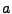is determined by the equation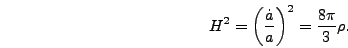(6.87)

Initiallyis set to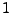and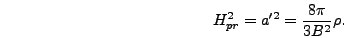(6.88)

In setting initial values we assume all inhomogeneities are small and thus use only the homogeneous values of the fields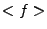and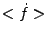. Typically the initial field values will be one for the inflaton and zero for all other fields but they can be set to any values by the user. In general, the initial energy density is thus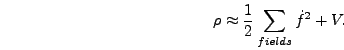(6.89)

Converting to program variables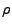(6.90)where the second step uses the fact that initially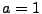. Since initially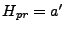we can plug equation (6.90) into equation (6.88) to get an equation we can solve for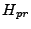. Solving this quadratic equation gives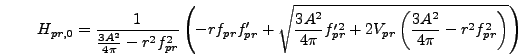(6.91)

where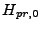refers to the initial value ofand each term with field or field derivative values is understood to be summed over all fields.Next: The Adiabatic Approximation Up: Initial Conditions on the Previous: Initial Conditions in One